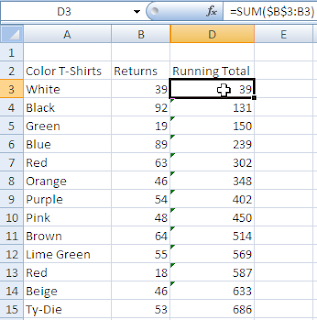## Thursday, February 25, 2010

### Cumulative Totals

Sometimes it is the easy stuff that escapes us.
Below is a screenshot showing how to create a cumulative total.

In the example below, I want to create a Running Total and track my T-Shirt Returns.
• Click in cell D3 and type =SUM(\$B\$3.B3)
• Press Enter
• Copy the formula down.Why this works:
The \$B\$3 creates an absolute cell reference so that as the formula is copied down, it always goes back and refers to B3.
If you click in cell D4, after you create the formula, you would see that the formula has now changed to
=SUM(\$B\$3:B4). The first cell reference is an absolute and anchors the cell reference in place but the second cell reference moves down a row.
The formula at D15 would of course read as =SUM(\$B\$3:B15).